20 Sample Fun Math Worksheet Templates

When it comes to educating little kids about maths and calculations, it’s smarter to take a fun way. Thus, you have the fun math worksheets(Addition and Subtraction) with images and cartoons that make calculations all the more interesting and playful for the young minds. Such a fun approach renders a light interesting quotient to education so that the kids are never bored to study. When it is fun to learn, education becomes more effective. If creating these fun worksheets every now and then is getting tiring in your packed schedule as a teacher, you can anyday go for the online sample fun math worksheets.

Colourful Fun Math Worksheet Template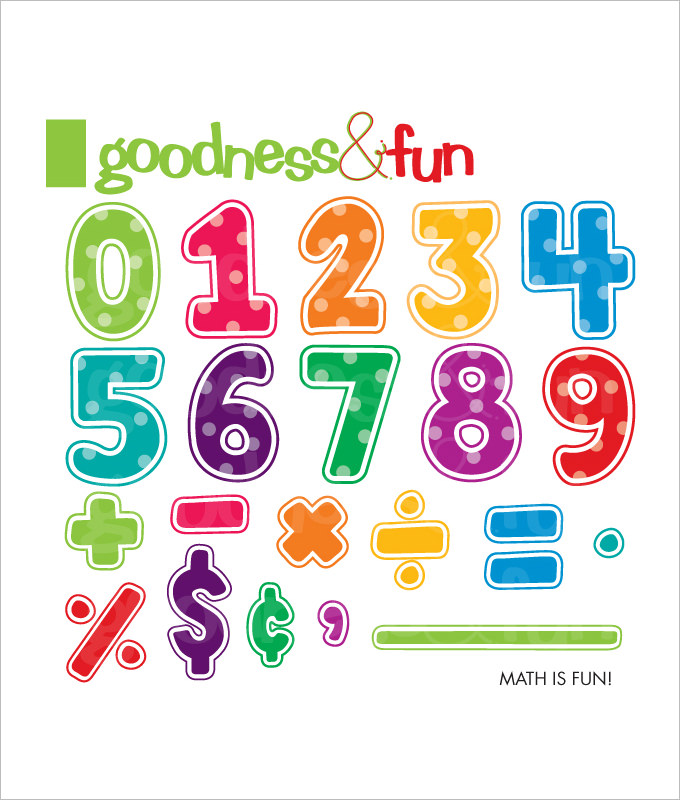Fun Multiplication Math Worksheet Template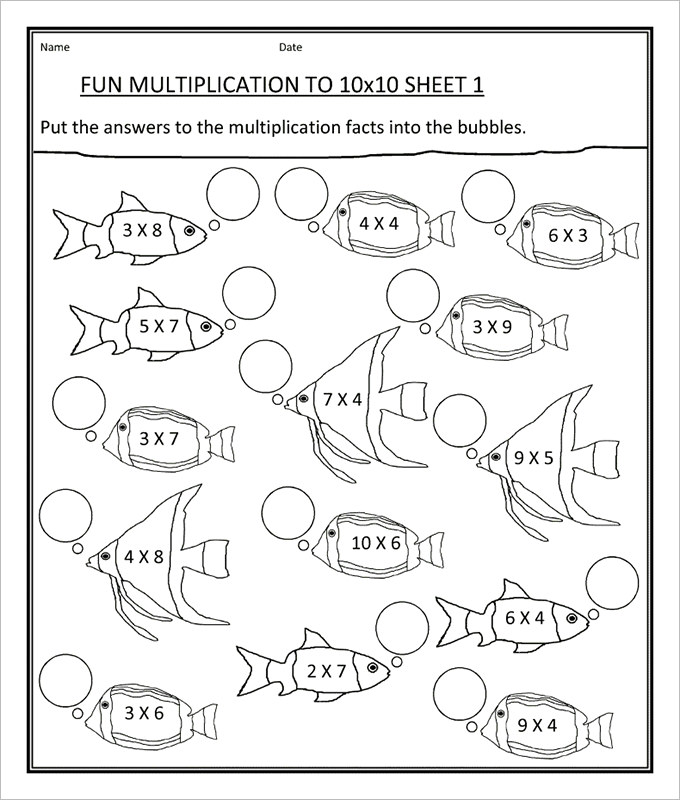Maze Fun Math Worksheet Template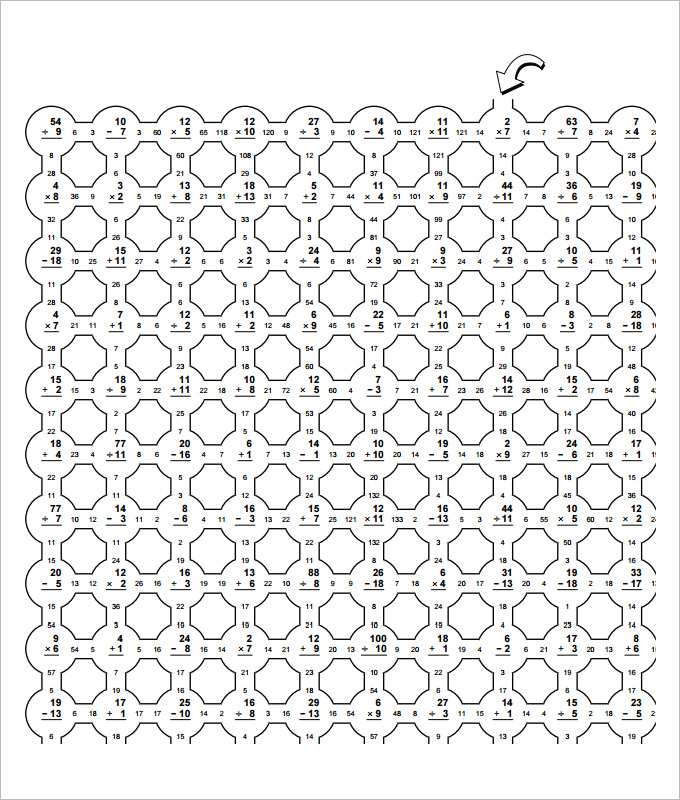Subtraction Maze Fun Math Worksheet Template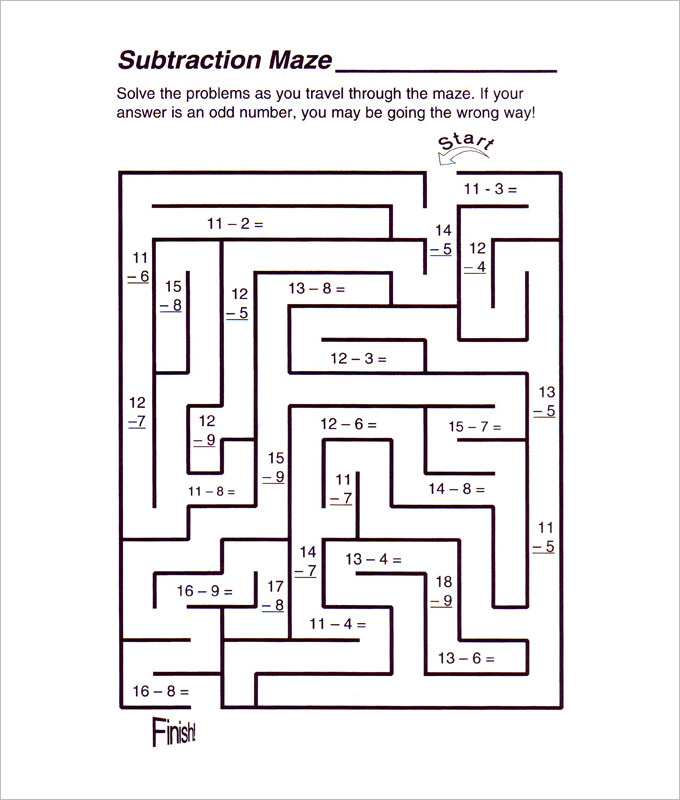Puzzle Fun Math Worksheet TemplateMore Number Fun Math Worksheet Template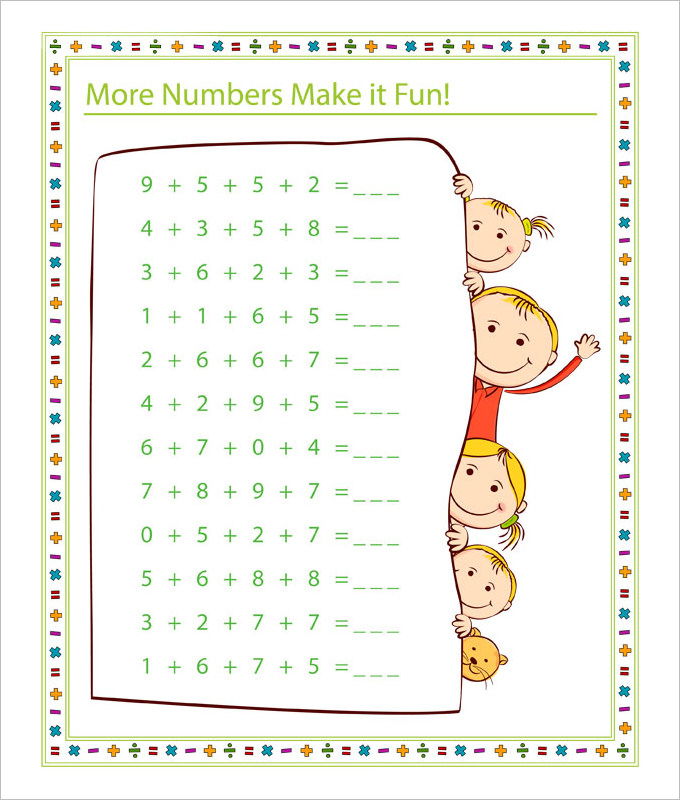Colouring Fun Math Worksheet Template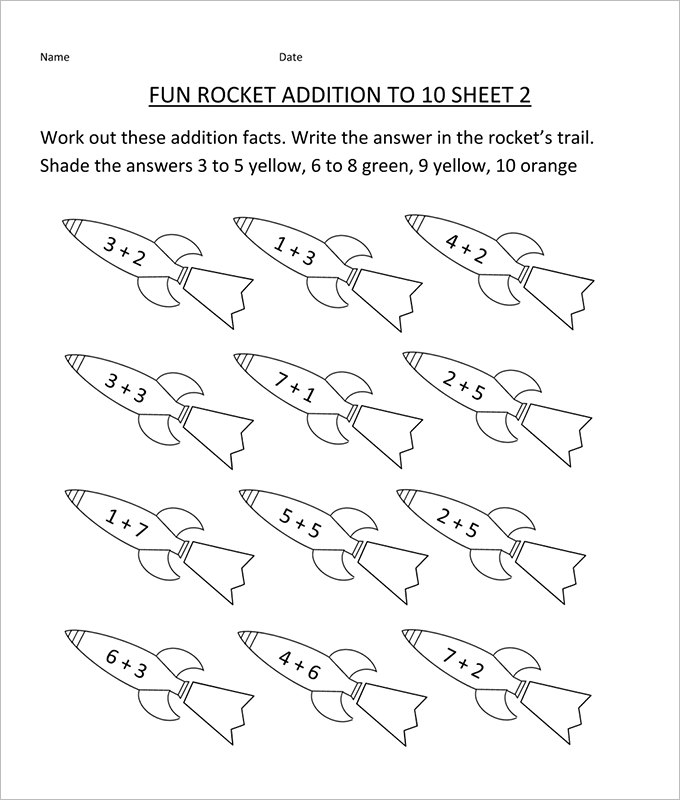Apple Fun Math Worksheet Template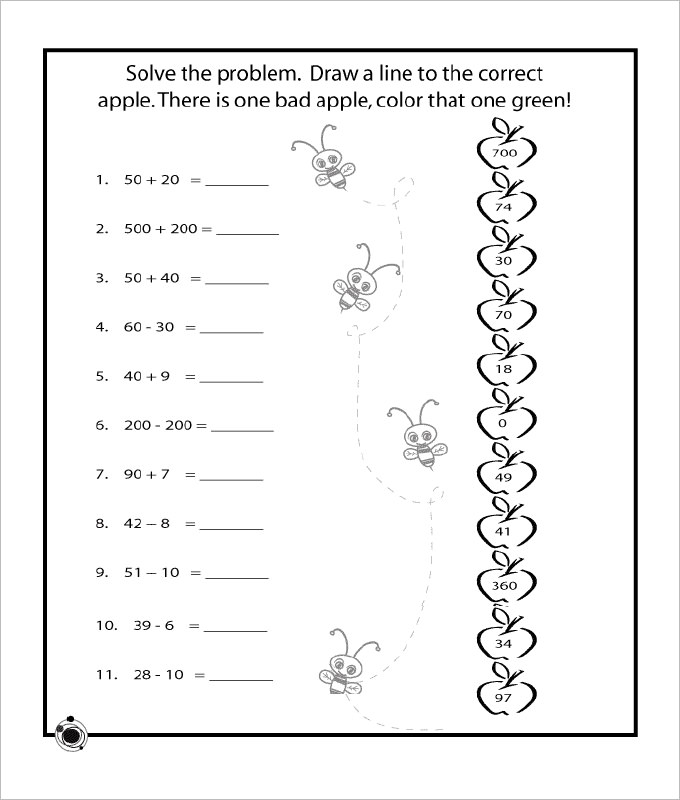Sample Fun Math Worksheet Template in PDF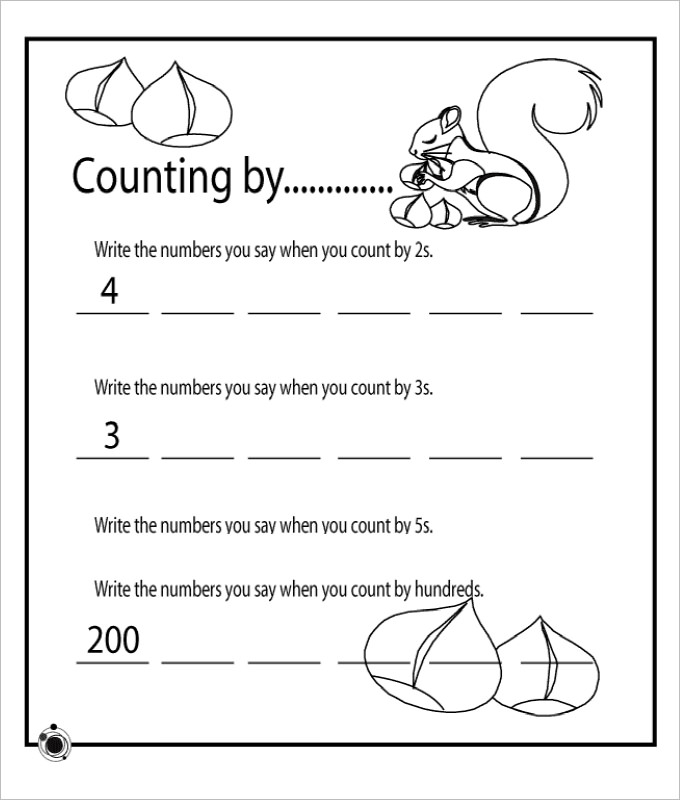Rounds Fun Math Worksheet Template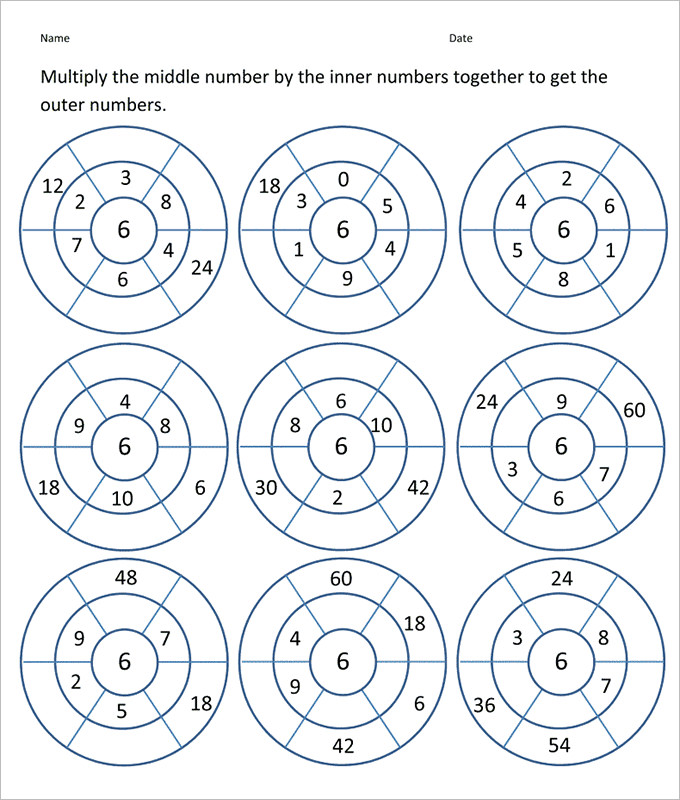Riddles Fun Math Worksheet Template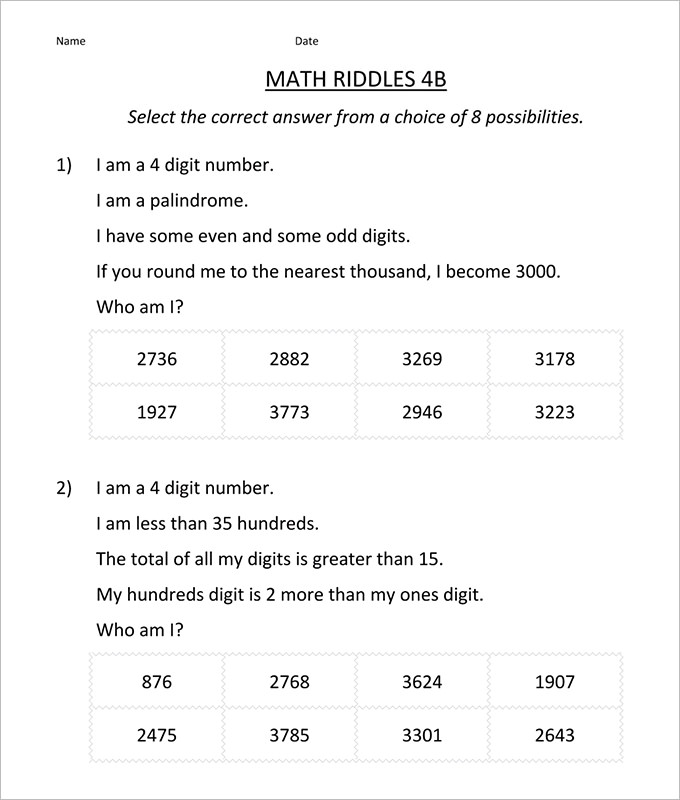Triangle Fun Math Worksheet Template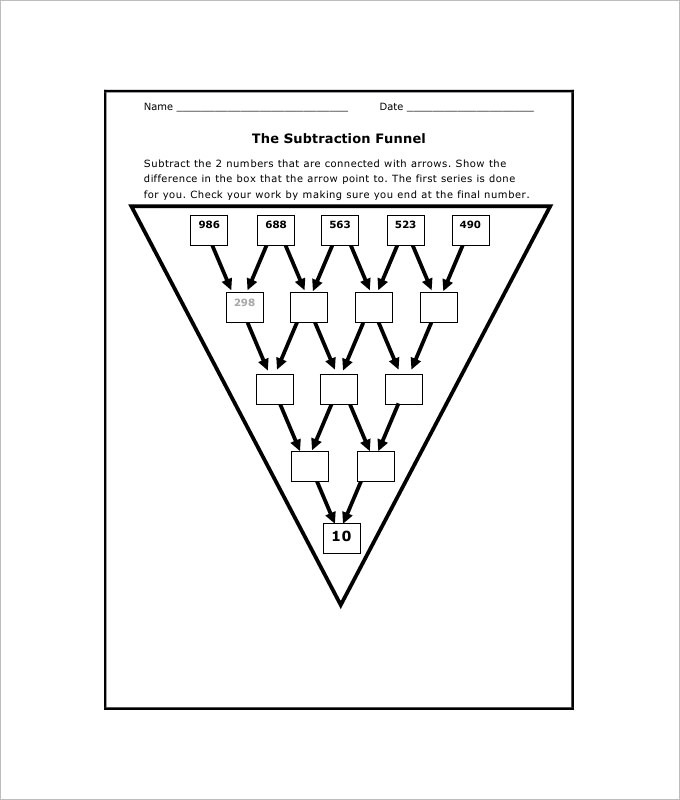Fun Hexagon Math Worksheet Template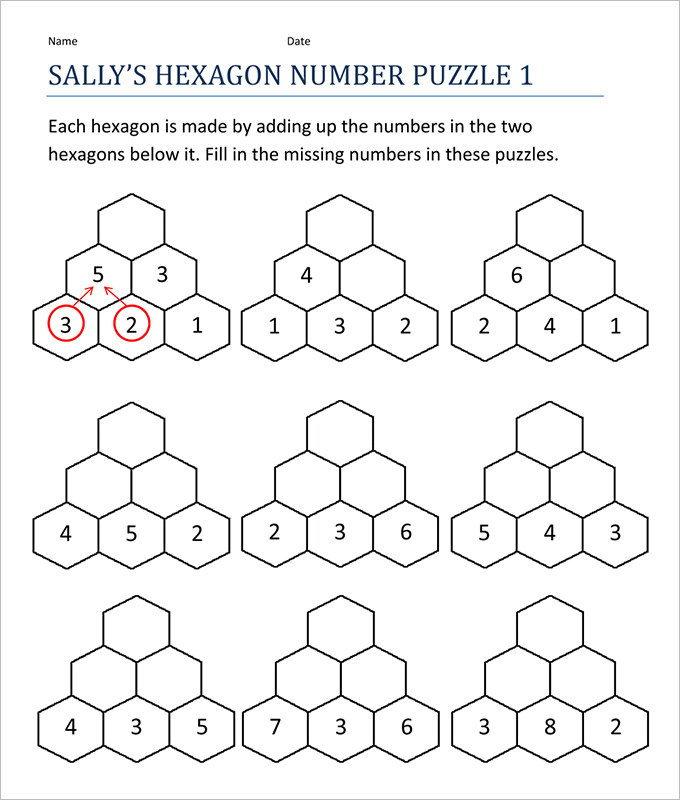Rounding Hundred Fun Math Worksheet Template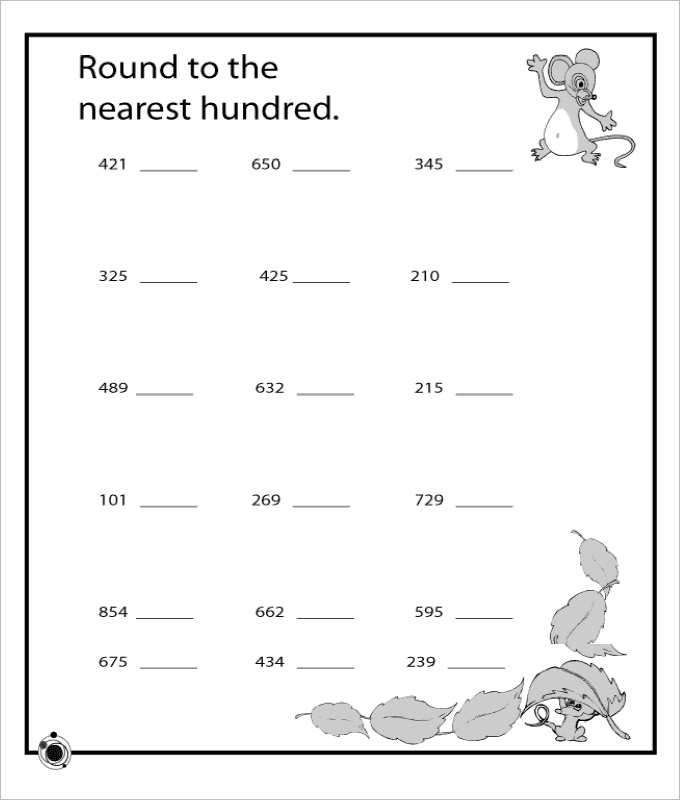Crossword Fun Math Worksheet Template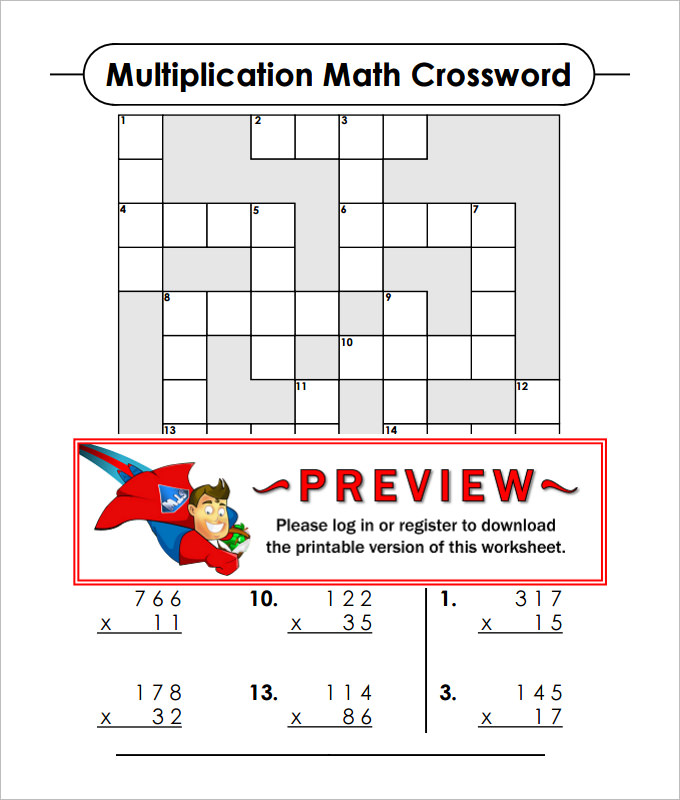Benefits of such online Fun Math Worksheets:

• You will get readymade worksheets here with pre-structured fun math activities so that you are no more needed to draft the worksheets yourself.
• There are different fun math activity sheets online to choose from such as missing numbers worksheets.
• You will even get interesting bar graph worksheets online with bars and cute pictures.
• You will be able to use the same worksheet for the entire class.
• These worksheets will save both time and energy for you.
• You have several sites over the internet that come up with fun math worksheets free download.

We have collected some Sample Fun Math Worksheet Templates, hope these templates will help you.

Sample Fun Math Worksheets | How to Make Fun Math Worksheet Fun Math Worksheets for Kids | Preschool Fun Math Worksheet Fun Multiplication Worksheets